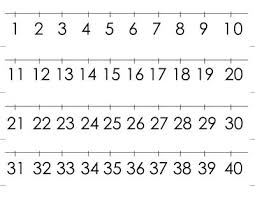# Consecutive 68424

The sum of five consecutive natural numbers is 75. What is the smallest of these numbers?

a =  13

### Step-by-step explanation:Did you find an error or inaccuracy? Feel free to write us. Thank you!

Tips for related online calculators
Do you have a linear equation or system of equations and looking for its solution? Or do you have a quadratic equation?